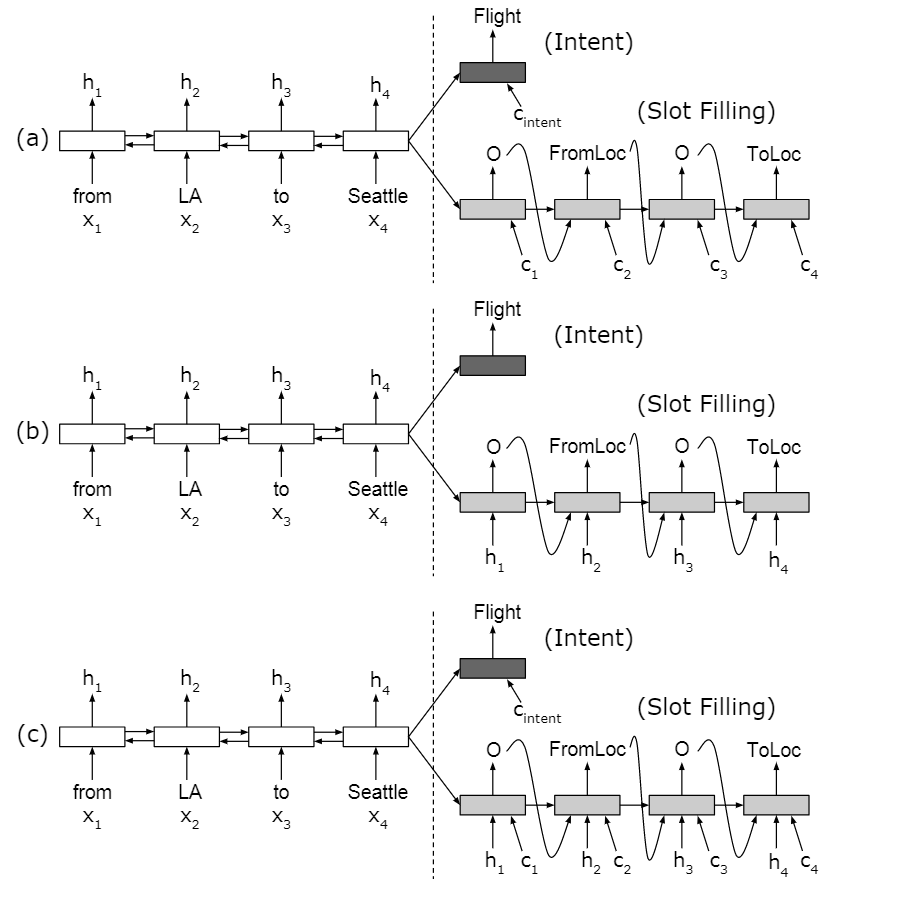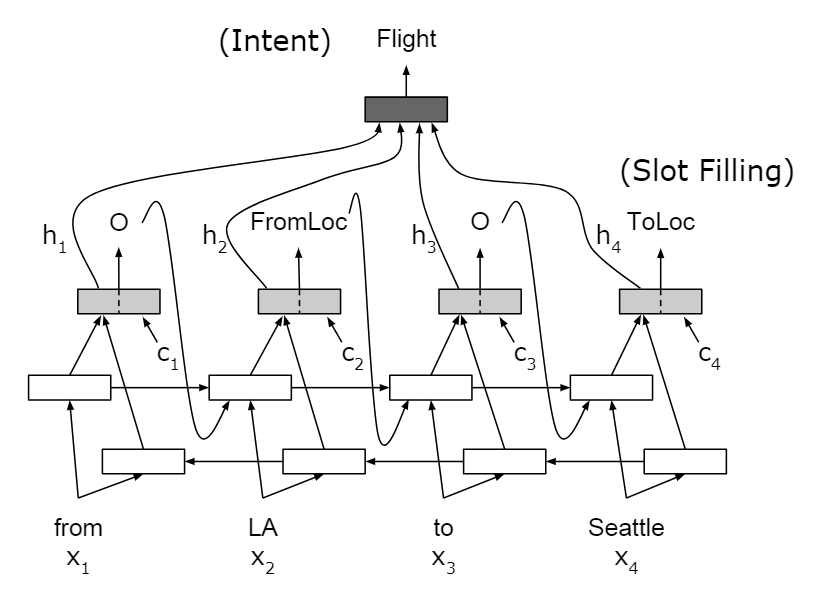# 背景

## 语义理解的两个方面

1. 语义理解的两个主要方面

• 理解说话人的意图 -- 意图检测（Intent Detection）
• 从句子中提取语义成分 -- 槽填充（Slot Filling）

2. 意图检测

3. 槽填充

4. 处理

5. 对齐和注意力

## 槽填充

1. 问题

Slots B-机舱类别 I-机舱类别 O O B-出发地 O B-目的地

2. RNN 槽填充

• $\mathbf x$ ：输入序列
• $\mathbf y$ ：输出序列
• $y_t$ ：第t个单词的slot lable

# 两种方法

## Seq2Seq方法

Encoder-Decoder with Aligned Inputs

1. 编码

2. 意图识别

3. 槽填充

1. 只有注意力输入

2. 只有对齐输入

3. 有注意力和对齐两个输入4. 带注意力和对齐输入的RNN槽填充计算方式 $s_0 = h_T$ 计算注意力的上下文$\mathbf c_i$ $\alpha_{ij} = \rm{softmax}(e_{ij})$

$e_{ij} = g(\mathbf s_{i-1}, \mathbf h_k)$

$\mathbf c_i = \sum_{j=1}^T\alpha_{ij} \mathbf h_j$

## 基于注意力的RNN